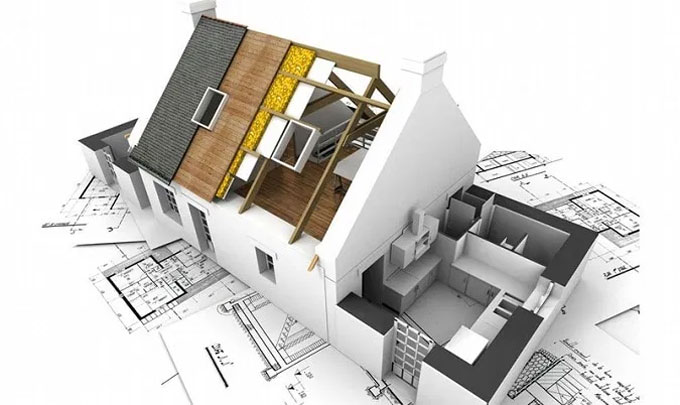# Most Common Measurement Units in ConstructionThe units of measurements (UOM) for civil engineering works are mainly categorized for their nature, shape and size and for making payments to the contractor and also. The principle of units of measurements normally consists of the following matters:

1. Single units work like doors, windows, trusses etc., are expressed in numbers.
2. Works consisting linear measurements involve length like cornice, fencing, hand rail, bands of specified width etc., are expressed in running meters (RM).

3. Works consisting areal surface measurements involve area like plastering, white washing, partitions of specified thickness etc., and are expressed in square meters (m2).
4. Works consisting cubical contents which involve volume like earth work, cement concrete, Masonry etc. are expressed in Cubic meters.

The rules for measurement of each item are invariably described in IS-1200. However, some of the general thumb rules are listed as following.

Measurement shall be made for finished item of work and description of each item shall include materials, transport, labor, fabrication tools and plant and all types of overheads for finished the work in required shape, size and specification.

In booking, the order shall be in sequence of length, breadth and height or thickness. All works shall be measured subject to the following tolerances:

1. Linear measurement shall be measured to the nearest 0.01m.
2. Areas shall be measured to the nearest 0.01 SQM
3. Cubic contents shall be worked out to the nearest 0.01 cum.

Same type of work under different conditions and nature shall be measured separately under separate items. The bill of quantities shall fully describe the materials, proportions, workmanships and accurately represent the work to be executed. In case of masonry (stone or brick) or structural concrete, the categories shall be measured separately and the heights shall be described as:

1. From foundation to plinth level
2. From plinth to first floor level
3. From first floor to second floor level and so on.

Common UoM in Construction - Following are some items of civil engineering work and their measuring unit:

a. Earth work in Excavation ? m3 (Cubic meter).
b. Earth work in Filing ? m3 (Cubic meter).
c. Brick work ? m3 (Cubic meter).
d. Plastering work ? m2 (Square meter).
e. Concrete work ? m3 (Cubic meter).
f. Reinforcement ? Kg (Kilogram).

g. Painting ? m2 (Square meter)
h. Skirting ? r.m. (running meter)
i. Flooring ? m2 (Square meter).
j. Brick work (4.5 ? thick) ? m2 (Square meter).
k. Structural steel work ? Kg (Kilogram).
l. Grills ? Kg (Kilogram) or m2 (Square meter).
m. Doors and windows ? m2 (Square meter).
n. Pointing work ? m2 (Square meter).
o. Retaining Wall ? m3 (Cubic meter).
p. M.S pipes for steel structure ? r.m. (running meter)
q. Water line, sewer line, drainage pipe ? r.m. (running meter)
r. Railing ? r.m. (running meter).
s. Soling work ? m2 (Square meter).
t. Manholes ? No. (Number).
u. Chambers ? No. (Number).
v. Plumbing and sanitary fittings work ? No. (Number).

Unit Conversion and Inter-unit Relation: Furthermore, you can use the following listing to quickly convert between the common units of measurements from one system to another. Also, the list will give you a basic idea about how the units are related to each other.

FEET / METERS

1 Feet = 12 inches
1 Feet = 0.3048 meter
1 Feet = 0.0929 meter square
1 Inch = 25.4 mm
1 Meter = 1,000 mm
1 Meter =3.281 feet
1 Meter = 1.094 yard
1 Meter Square = 10.764 square feet

CENTIMETER

1 Centimeter = 0.3937 inches
1 Centimeter = 0.032808 feet
2.54 Centimeter= 1 inch
30.48 Centimeters = 1 Foot
91.44 Centimeters = 1 yard

ACRE

1 Acre = 43,560 Square Feet
1 Acre = 160 square rods
1 Acre = 10 square chains
1 Acre = 160 poles
1 Acre = 0.4047 Hectare
1 Acre = 100 square meters

HECTARE

1 Hectare = 10,000 Square meter
1 Hectare = 107,639 sq ft
1 Hectare = 11959.8 sq. yards
1 Hectare = 11959.8/4840 = 2.47 acre

FOOT / FURLONG

1 Foot = 12 inches
1 Foot = 0.36 vars
1 Foot = 0.3048006
1 Furlong = 660 feet
1 Furlong =40 rods

MILE

1 Mile = 5280 feet
1 Mile =8 furlong
1 Mile = 320 rods
1 Mile = 80 chains
1 Mile = 1.60935 kilometers
1 Mile = 320 perches
1 Mile = 1609.2655 meters
1 Mile Square = 27,878,400 square feet
1 Mile Square = 2.59 square hectares

YARD

1 Yard = 36 inches
1 Yard = 3 feet
1 yard = 0.9144 meters
1 Yard = 91.44 centimeter
1 Square Yard = 9 square feet